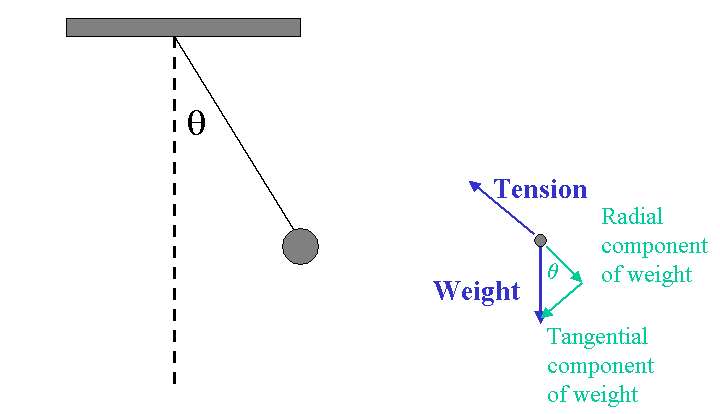# Component forces of a pendulum

yucheng
http://www1.lasalle.edu/~blum/p106wks/pl106_Pendulum.htm#:~:text=The forces acting on the,the tension of the string.&text=The net radial force leads,is v2/r.)

P.S. I'll insert my specific questions in the following paragraphs in this format: {{Q(reference number):}}.
P.S.S I'll use the xy coordinate system (horizontal and vertical), also the radial and tangential coordinate system.I am referring to the diagram above. Resolving weight into its components, the following equation was given: $$T-mg\cos\theta=ma_{radial}\tag1$$ I was wondering why is the equation true? Let me try by resolving the vector for tension. $$T_y=T\cos\theta \tag2$$ $$T_y=T\sin\theta \tag3$$ Let's assme $$T-mg\cos\theta>0$$, as if it is zero, this means that the $$weight > T$$ and the string will break. {{Q1: Is this reasoning acceptable?}}

Now, let's backtrack a bit and change the way we approached the problem. Instead of resolving weight into its components, we equate ##\tag1## with weight because otherwise, the string will break if ##w>(2)##, and the pendulum bob will not remain in circular motion if there is a component along the vertical axis ##w<(2)## {{Q2: Is this reasoning acceptable?}}. Does this mean that $$T_x=a_{radial}$$, given that the pendulum only moves along the circle in a plane, thus the change in direction is only caused by ##T_x## which is in the same plane? Well, at least that's what my textbook tells me.

Back to our original question, on equation ## (1)##, and given our reasoning on why (1) must be greater than 0, this means we can further resolve (1) into its components? Suppose we equate (1) with ##T"##, however, ##T'## does have a vertical component. The reason being there is still the tangential component of weight, and thus a vertical component of weight which (1) does not take into account. Considering our argument above how the ##a_{radial}## does not have a vertical component, how is ##T'=ma_{radial}##? {{Q3}}

Last edited: